x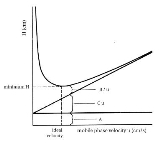Van Deemter's equationEncyclopedia
The Van Deemter equation in chromatography
Chromatography
Chromatography is the collective term for a set of laboratory techniques for the separation of mixtures....

relates the variance per unit length of a separation column to the linear mobile phase velocity
Velocity
In physics, velocity is speed in a given direction. Speed describes only how fast an object is moving, whereas velocity gives both the speed and direction of the object's motion. To have a constant velocity, an object must have a constant speed and motion in a constant direction. Constant ...

by considering physical, kinetic, and thermodynamic properties of a separation. These properties include pathways within the column, diffusion
Diffusion
Molecular diffusion, often called simply diffusion, is the thermal motion of all particles at temperatures above absolute zero. The rate of this movement is a function of temperature, viscosity of the fluid and the size of the particles...

(axial
Axial
Axial may mean:* Along the same line as an axis of rotation in geometry* A type of modal frame in music* One of several anatomical directions in an animal body* Axial age, the period from 800 to 200 BC in China, India and the western world...

and longitudinal), and mass transfer
Mass transfer
Mass transfer is the net movement of mass from one location, usually meaning a stream, phase, fraction or component, to another. Mass transfer occurs in many processes, such as absorption, evaporation, adsorption, drying, precipitation, membrane filtration, and distillation. Mass transfer is used...

kinetics
Chemical kinetics
Chemical kinetics, also known as reaction kinetics, is the study of rates of chemical processes. Chemical kinetics includes investigations of how different experimental conditions can influence the speed of a chemical reaction and yield information about the reaction's mechanism and transition...

between stationary and mobile phases. In liquid chromatography, the mobile phase velocity is taken as the exit velocity, that is, the ratio of the flow rate in ml/second to the cross-sectional area of the ‘column-exit flow path.’ For a packed column, the cross-sectional area of the column exit flow path is usually taken as 0.6 times the cross-sectional area of the column. Alternatively, the linear velocity can be taken as the ratio of the column length to the dead time. If the mobile phase is a gas, then the pressure
Pressure
Pressure is the force per unit area applied in a direction perpendicular to the surface of an object. Gauge pressure is the pressure relative to the local atmospheric or ambient pressure.- Definition :...

correction must be applied. The variance per unit length of the column is taken as the ratio of the column length to the column efficiency in theoretical plate
Theoretical plate
A theoretical plate in many separation processes is a hypothetical zone or stage in which two phases, such as the liquid and vapor phases of a substance, establish an equilibrium with each other. Such equilibrium stages may also be referred to as an equilibrium stage, ideal stage or a theoretical...

s. The Van Deemter equation is a hyperbolic function
Hyperbolic function
In mathematics, hyperbolic functions are analogs of the ordinary trigonometric, or circular, functions. The basic hyperbolic functions are the hyperbolic sine "sinh" , and the hyperbolic cosine "cosh" , from which are derived the hyperbolic tangent "tanh" and so on.Just as the points form a...

that predicts that there is an optimum velocity at which there will be the minimum variance per unit column length and, thence, a maximum efficiency. The Van Deemter equation was the result of the first application of rate theory to the chromatography elution process.

## Van Deemter equation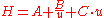Where
• A = Eddy-diffusion
• B = Longitudinal diffusion
• C = mass transfer
Mass transfer
Mass transfer is the net movement of mass from one location, usually meaning a stream, phase, fraction or component, to another. Mass transfer occurs in many processes, such as absorption, evaporation, adsorption, drying, precipitation, membrane filtration, and distillation. Mass transfer is used...

kinetics of the analyte between mobile and stationary phase
• u = Linear Velocity.

A is equal to the multiple paths taken by the chemical compound
Chemical compound
A chemical compound is a pure chemical substance consisting of two or more different chemical elements that can be separated into simpler substances by chemical reactions. Chemical compounds have a unique and defined chemical structure; they consist of a fixed ratio of atoms that are held together...

, in open tubular
Tubular
Tubular may refer to:*the form of a cylinder or tube*Tubular, a television-related entertainment blog on the Houston Chronicle website*Tubular, a level in the video game Super Mario World...

capillaries
Capillary
Capillaries are the smallest of a body's blood vessels and are parts of the microcirculation. They are only 1 cell thick. These microvessels, measuring 5-10 μm in diameter, connect arterioles and venules, and enable the exchange of water, oxygen, carbon dioxide, and many other nutrient and waste...

this term will be zero as there are no multiple paths. The multiple paths occur in packed columns where several routes exist through the column packing, which results in band spreading.

B/u is equal to the longitudinal diffusion of the particles of the compound.

Cu is equal to the equilibration point. In a column, there is an interaction between the mobile and stationary phases, Cu accounts for this.

A minimum value for H can be found by differentiating: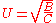## Plate count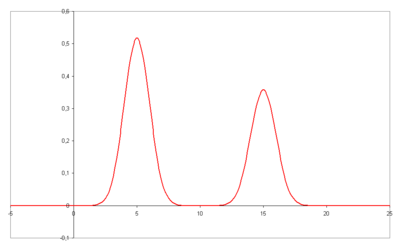The plate height given as: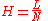with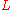the column length and :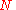the number of theoretical plates can be estimated from a chromatogram by analysis of the retention time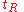for each component and its standard deviation
Standard deviation
Standard deviation is a widely used measure of variability or diversity used in statistics and probability theory. It shows how much variation or "dispersion" there is from the average...as a measure for peak width, provided that the elution curve represents a Gaussian curve.

In this case the plate count is given by: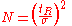By using the more practical peak width at half height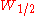the equation is: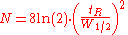or with the width at the base of the peak: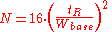## Expanded van Deemter

The Van Deemter equation can be further expanded to: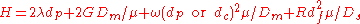Where:
• H is plate height
• λ is particle shape (with regard to the packing)
• dp is particle diameter
• G, ω, and R are constants
• Dm is the diffusion coefficient of the mobile phase
• dc is the capillary diameter
• df is the film thickness
• Ds is the diffusion coefficient of the stationary phase.

Van Deemter's equation indicates that band broadening mechanisms are proportionally dependent on flow rate, inversely proportional to flow rate and independent of flow rate.